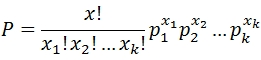# SQL Server Mulinomial Probability function

MULTINOM
Updated: 30 May 2017

Use the SQL Server aggregate function MULTINOM to calculate the probability distribution of the outcomes of a multinomial experiment. Use MULTINOM for statistical experiments where:

• The experiment consists of x repeated trials.
• Each trial has a discrete number of possible outcomes.
• On any given trial, the probability that a particular outcome will occur is constant
• The trials are independent; that is, the outcome on one trial does not affect the outcome on other trials.Syntax
SELECT [wct].[MULTINOM] (
<@x, int,>
,<@p, float,>)
Arguments
 Input Name Definition @x The bin value for the trials @p The probability for that bin
Return Type
float
Remarks
• If @p is NULL then @p = 1.
• If @x is NULL then it is not included in the calculation.
• p is internally normalized so that SUM(@p) = 1.
• x! is the factorial of the sum of @x
• Available in XLeratorDB / statistics 2008 only
Examples
Example #1

Suppose we have an urn containing 9 marbles. Two are red, three are green, and four are blue. 5 marbles are randomly selected from the urn with replacement. What is the probability of selecting 2 green marbles and 3 blue marbles?

SELECT
wct.MULTINOM(x,p) as MULTINOM
FROM (VALUES
('red',0,2)
,('green',2,3)
,('blue',3,4)
)n(c,x,p)

This produces the following result.

Example #2

Suppose we roll a dice 5 times and obtain three ones and two sixes; in other words, a 6-tuple of (3,0,0,0,0,2). We can calculate the probability with the following SQL.

SELECT
wct.MULTINOM(x,p) as MULTINOM
FROM (VALUES
(3,NULL)
,(0,NULL)
,(0,NULL)
,(0,NULL)
,(0,NULL)
,(2,NULL)
)n(x,p)

This produces the following result.Copyright 2008-2021 Westclintech LLC         Privacy Policy        Terms of Service